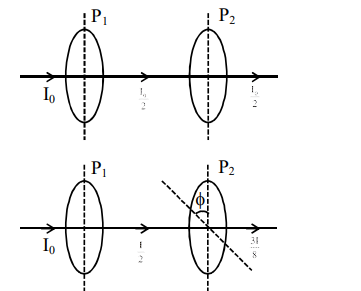# A source of light is placed in front of a screen.Question:

A source of light is placed in front of a screen. Intensity of light on the screen is I. Two Polaroids $P_{1}$ and $P_{2}$ are so placed in between the source of light and screen that the intensity of light on screen is $\mathrm{I} / 2 . \mathrm{P}_{2}$ should be rotated by an angle of (degrees) so that the intensity of light on the screen becomes $\frac{3 \mathrm{I}}{8}$.

Solution:

$I=\frac{I_{0}}{2} \cos ^{2} \phi$$\frac{I}{2} \cos ^{2} \phi=\frac{3 I}{8}$

$\cos ^{2} \phi=\frac{3}{4}$

$\cos ^{2} \phi=\frac{\sqrt{3}}{2}$

$\Rightarrow \phi=30$

Ans. 30